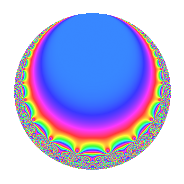# Properties

 Label 16.5.cLevel 16 Weight 5 Character orbit c Rep. character $$\chi_{16}(15,\cdot)$$ Character field $$\Q$$ Dimension 2 Newforms 1 Sturm bound 10 Trace bound 0

# Related objects

## Defining parameters

 Level: $$N$$ = $$16 = 2^{4}$$ Weight: $$k$$ = $$5$$ Character orbit: $$[\chi]$$ = 16.c (of order $$2$$ and degree $$1$$) Character conductor: $$\operatorname{cond}(\chi)$$ = $$4$$ Character field: $$\Q$$ Newforms: $$1$$ Sturm bound: $$10$$ Trace bound: $$0$$

## Dimensions

The following table gives the dimensions of various subspaces of $$M_{5}(16, [\chi])$$.

Total New Old
Modular forms 11 2 9
Cusp forms 5 2 3
Eisenstein series 6 0 6

## Trace form

 $$2q$$ $$\mathstrut +\mathstrut 36q^{5}$$ $$\mathstrut -\mathstrut 222q^{9}$$ $$\mathstrut +\mathstrut O(q^{10})$$ $$2q$$ $$\mathstrut +\mathstrut 36q^{5}$$ $$\mathstrut -\mathstrut 222q^{9}$$ $$\mathstrut +\mathstrut 356q^{13}$$ $$\mathstrut -\mathstrut 252q^{17}$$ $$\mathstrut +\mathstrut 768q^{21}$$ $$\mathstrut -\mathstrut 602q^{25}$$ $$\mathstrut -\mathstrut 2844q^{29}$$ $$\mathstrut +\mathstrut 3456q^{33}$$ $$\mathstrut +\mathstrut 1060q^{37}$$ $$\mathstrut +\mathstrut 324q^{41}$$ $$\mathstrut -\mathstrut 3996q^{45}$$ $$\mathstrut +\mathstrut 3266q^{49}$$ $$\mathstrut +\mathstrut 1188q^{53}$$ $$\mathstrut -\mathstrut 11136q^{57}$$ $$\mathstrut +\mathstrut 1252q^{61}$$ $$\mathstrut +\mathstrut 6408q^{65}$$ $$\mathstrut +\mathstrut 20736q^{69}$$ $$\mathstrut -\mathstrut 13372q^{73}$$ $$\mathstrut -\mathstrut 6912q^{77}$$ $$\mathstrut -\mathstrut 6462q^{81}$$ $$\mathstrut -\mathstrut 4536q^{85}$$ $$\mathstrut +\mathstrut 16452q^{89}$$ $$\mathstrut -\mathstrut 9216q^{93}$$ $$\mathstrut -\mathstrut 3196q^{97}$$ $$\mathstrut +\mathstrut O(q^{100})$$

## Decomposition of $$S_{5}^{\mathrm{new}}(16, [\chi])$$ into irreducible Hecke orbits

Label Dim. $$A$$ Field CM Traces $q$-expansion
$$a_2$$ $$a_3$$ $$a_5$$ $$a_7$$
16.5.c.a $$2$$ $$1.654$$ $$\Q(\sqrt{-3})$$ None $$0$$ $$0$$ $$36$$ $$0$$ $$q-\zeta_{6}q^{3}+18q^{5}+2\zeta_{6}q^{7}-111q^{9}+\cdots$$

## Decomposition of $$S_{5}^{\mathrm{old}}(16, [\chi])$$ into lower level spaces

$$S_{5}^{\mathrm{old}}(16, [\chi]) \cong$$ $$S_{5}^{\mathrm{new}}(4, [\chi])$$$$^{\oplus 3}$$